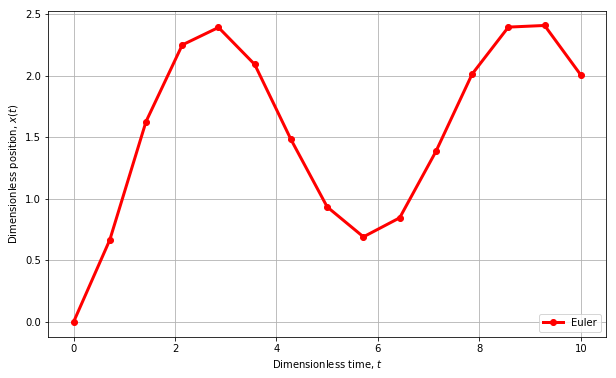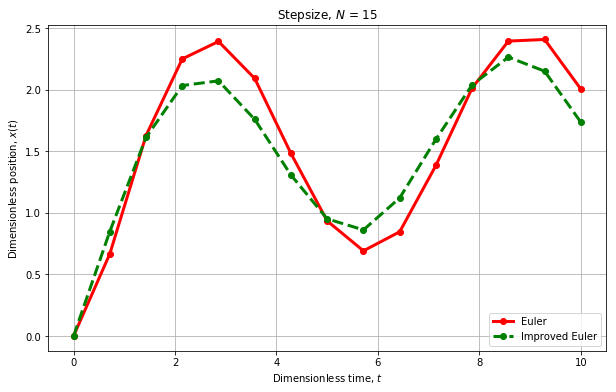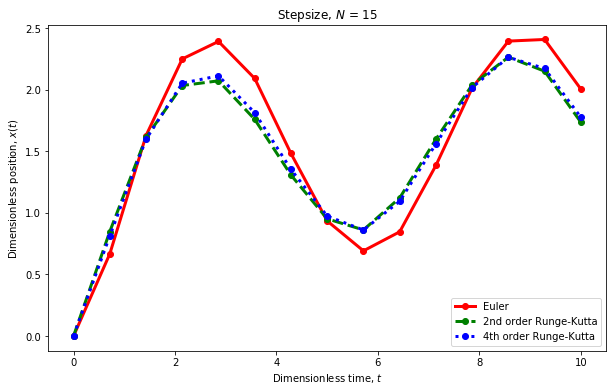# The Runge-Kutta Method¶

### Modules - Ordinary Differential Equations¶

Last edited: February 7th 2018

In other notebooks we've studied Euler's method for solving ordinary differential equations (ODEs). This method is perhaps the most simple to understand and implement and thus is very popular as teaching material. However, for scientific purposes, Euler's method is inaccurate compared to more complex methods using the same step size, and are also conditionally unstable as discussed the previous modules.

Here, we'll consider the Runge-Kutta Method, which is one of the most applied numerical methods for solving ODEs. It is deemed reasonably efficient considering computation time and its fourth order approximation offers decent accuracy, that is, good enough for most problems not demanding solutions of very high precision. Do note that we present the method in its simplest form: You may encounter its implementation somewhat different elsewhere. In general, newer (and smarter) implementations of the method can provide significantly boosts to its efficiency.

## Revisiting Euler's Method¶

Consider the the same (non-linear) ODE as discussed in the previous modules,

$$\dot{x}(t) = \cos(x(t)) + \sin(t), \qquad \dot{x}\equiv\frac{\textrm{d}x(t)}{\textrm{d}t}$$

with initial condition $x(t_0) = 0, t_0 = 0$. The (Explicit) Euler method solves this problem by discretizing the variables such that

\begin{align*} t & \rightarrow t_n \qquad\equiv t_0 + n\cdot\Delta t,\\[1.0em] x(t) & \rightarrow x(t_n) \quad\equiv x_n ,\\[1.0em] n & = 0,1,\ldots,N; \end{align*}

Then, the function value $x_{n+1}$ may be approximated by the preceding value plus the change (derivative) in that point multiplied with the distance in time $\Delta t$ between $x_n$ and $x_{n+1}$. That is,

$$x_{n+1} = x_n + (\Delta t) \cdot \dot{x}_n, \qquad \Delta t = \frac{t_N - t_0}{N} \equiv h$$

For this particular problem, we are given $\dot{x}_n(t_n)$, such that this can be inserted directly into the Euler formula giving,

$$x_{n+1} = x_n + h [\cdot \cos(x_n) + \sin(t_n)]$$

Which is about everything needed to solve the current problem. For a more thorough and detailed explaination of the Euler method, take a look at the modules 4.1 (explicit) and 4.3 (implicit)

In :
%matplotlib inline
import numpy as np               # Numerical Python
import matplotlib.pyplot as plt  # Graph and Plot handling
import time                      # Time Measure

In :
# A quick implementation of Euler's Method for solving
# the above Initial Value Problem

# Try adjusting 'N' to see how the numerical solution converges

t0   = 0.0
tN   = 10.0
N    = 15
t    = np.linspace(t0,tN,N)
x_Eu = np.zeros(N) ## Euler
h    = (tN-t0)/N

# Initial condition:
x_Eu = 0

for n in range(0,N-1):
x_Eu[n+1] = x_Eu[n] + h * (np.cos(x_Eu[n]) + np.sin(t[n]))

plt.figure(figsize=(10,6))
plt.plot(t,x_Eu,'-ro',linewidth=3.0, label=r'Euler')
plt.ylabel(r'Dimensionless position, $x(t)$')
plt.xlabel(r'Dimensionless time, $t$')
plt.legend(loc=4) # 'loc' sets the location of the legend-text in the plot
plt.grid()
plt.show()A quick error analysis shows that the local truncation error emitted in each and every step of Euler's method is proportional to the step size squared $h^2 = (\Delta t)^2$, by Taylor expanding the function about $t+h$

\begin{align*} \text{Exact:} & \qquad x(t + h) = x(t) + h\dot{x} + \frac{h^2}{2}\ddot{x} + \frac{h^3}{6}\dddot{x} + \ldots \\[1.2em] \text{Euler:} & \qquad x(t + h) \approx x(t) + h\dot{x}\\[1.2em] \text{Error:} & \qquad e = \frac{h^2}{2}\ddot{x} + \frac{h^3}{6}\dddot{x} + \ldots \end{align*}

Now, if we iterate the system a total of $N=\frac{t_N - t_0}{h}\propto h^{-1}$ times, then the total, global error is $E = N\cdot e \propto h$. That is, the Euler is a first order method, as concluded in the previous modules which also gives a more in-depth error analysis of the Euler method. The question now is: Can we obtain a better approximated solution of same step size?

## Improving Euler's Method¶

The underlying concept of the Runge-Kutta method we wish to show, is built on the same basic concepts as from Euler's method. Consider now that we apply Euler's method again, however this time we will not use the derivative at $x_{n+1}$, but rather at the middle point $x_{m}=\frac{x_{n+1}+x_n}{2}$, refered to as a test-point. One can then compute a more accurate $x_{n+1}$ using the information of the derivative at this midpoint ($\dot{x}_m$) of the interval $h$ between $x_n$ and $x_{n+1}$. That is,

\begin{align*} x_{n+1} &= x_n + h\cdot \dot{x}_m \\[1.2em] &= x_n + h\cdot [\cos(x_m) + \sin(t_m)] \\[0.8em] &= x_n + h\cdot \left[\cos\left(x_n + \frac{h \dot{x}_n}{2}\right) + \sin\left(t_n+\frac{h}{2} \right)\right] \\[1.0em] &= x_n + h\cdot \left[\cos\left(x_n + \frac{h}{2}[\cos(x_n)+\sin(t_n)]\right) + \sin\left(t_n+\frac{h}{2} \right)\right] \end{align*}

Now, let us plot it against the original Euler-approach and study the difference.

In :
x_imp = np.zeros(N)

for n in range(0,N-1):
x_imp[n+1] = x_imp[n] + h * ( np.cos(x_imp[n]+(h/2.0)*(np.cos(x_imp[n]) + np.sin(t[n]))) + np.sin(t[n]+h/2.0)  )

plt.figure(figsize=(10,6))
plt.plot(t,x_Eu ,'-ro' ,linewidth=3.0,label=r'Euler')
plt.plot(t,x_imp,'--go',linewidth=3.0,label=r'Improved Euler')
plt.ylabel(r'Dimensionless position, $x(t)$')
plt.xlabel(r'Dimensionless time, $t$')
plt.title(r'Stepsize, $N$ = %i' % N)
plt.legend(loc=4) # 'loc' sets the location of the legend-text in the plot
plt.grid()
plt.show()The new approximation is significantly different from the solution provided by the original Euler-approach (using $N=15$). What we have implemented here, is often referred to as The Improved Euler Method or The Midpoint Method for ODEs. However, a dear child has many names, and we will refer to it as The Second Order Runge-Kutta Method. If we assume our improved method to be more accurate, one sees that the original approach over-shoots, which is a known issue for the Euler method.

Let us in a short manner compare the errors of the two approaches, by first estimating the error of our improved scheme in a similar fashion as above, by Taylor expanding about $(t+h/2)$. The improved scheme applies the approximation

$$x(t+h) \approx x(t) + h\dot{x}(t+h/2)$$

where we expand $\dot{x}(t+h/2)$ resulting in

$$\dot{x}(t+h/2) = \dot{x}(t) + (h/2)\ddot{x} + \frac{(h/2)^2}{2}\dddot{x} + \mathcal{O}(h^3)$$

In a similar fashion as for the Euler method, we compare our improved scheme with the exact Taylor expansion,

\begin{align*} \text{Exact:} & \qquad x(t + h) = x(t) + h\dot{x} + \frac{h^2}{2}\ddot{x} + \frac{h^3}{6}\dddot{x} + \ldots \\[1.2em] \text{Impr. E.:} & \qquad x(t + h) \approx x(t) + h[\dot{x}(t) + (h/2)\ddot{x}]\\[1.2em] \text{Error:} & \qquad e = \frac{h^3}{6}\dddot{x} + \mathcal{O}(h^4) \end{align*}

This implies a global error $E=N\cdot e \propto h^2$, which effectivley gives a method of second order accuracy in $h$, not so surprising considering the name of the method. Let us ask ourselfs again: can we do it even better?

## The General (Fourth Order) Runge-Kutta Method¶

What Euler did was estimating $x_{n+1}$ by using $x_n$ and $\dot{x}_n$. What we just did, was estimating $x_{n+1}$ by using $x_n$ and the derivative of the point in-between, namely the mid-test-point, $x_m$. Now, here's an interesting idea: Would it be possible to use a similar test-point, to calculate the derivative to the actual test-point? Take some time to think about what this question implies before you read on.

The perhaps not so ground breaking answer to the above question is yes. In fact, we can do even better: We can make a test-point, for the test-point's test point, and so on! As it happens to be, The Fourth Order Runge-Kutta Method uses three such test-points and is the most widely used Runge-Kutta Method. You might ask why we don't use five, ten or even more test-points, and the answer is quite simple: It is not computationally free to calculate all these test-points, and the gain in accuracy rapidly decreases beyond the fourth order of the method. That is, if high precision is of such importance that you would require a tenth-order Runge-Kutta, then you're better off reducing the step size $h$, than increasing the order of the method.

Also, there exists other more sophisticated methods which can be both faster and more accurate for equivalent choices of $h$, but obviously, may be a lot more complicated to implement. See for instance Richardson Extrapolation, the Bulirsch-Stoer method, Multistep methods, Multivalue methods and Predictor-Corrector methods.

Nevertheless, we now show a general expression for the arbitrarily-ordered Runge-Kutta Method, before we apply the fourth order method on the problem given above. Again, consider an ODE written on the form

$$\dot{x}(t) = g(x(t),t)$$

Then, for the general $q$-ordered Runge-Kutta method, one has

\begin{align*} k_1 &= h\cdot g(x_n, t_n) \\[1.0em] k_2 &= h\cdot g(x_n + a_{2,1}k_1 , t+c_2 h ) \\[1.0em] k_3 &= h\cdot g(x_n + a_{3,1}k_1 + a_{3,2}k_2 , t+c_3 h ) \\[1.0em] k_4 &= h\cdot g(x_n + a_{4,1}k_1 + a_{4,2}k_2 + a_{4,3}k_3 , t+c_4 h ) \\[1.0em] &\qquad \vdots \\[1.0em] k_q &= h\cdot g(x_n + [a_{q,1} k_1 + a_{q,2}k_2+\ldots+a_{q,q-1}k_{q-1}], t_n + c_q h ) \end{align*}

Such that,

\begin{equation*} x_{n+1} = x_n + \sum_{i=1}^{q} b_i k_i \end{equation*}

The scheme as introduced now in its general form, has some undefined coefficients: $a_{i,j}$, $b_{i}$ and $c_{i}$. The elements $a_{i,j}$ are denoted as the Runge-Kutta Matrix, while $b_i$ are weights and $c_i$ are nodes. Deriving these (and coefficients for other similar methods) can be a tedious task of complicated algebra. Hence, such coefficients are usually obtained from tables in litterature. The Runge-Kutta methods' coefficients can be found using the (John C.) Butcher tableau,

\begin{array}{ c|c c c } 0 & && &&& \\ c_2 & a_{2,1} && &&& \\ c_3 & a_{3,1} && a_{3,2} &&& \\ \vdots & &\ddots& &&& \\ c_q & a_{q,1} && a_{q,2} & \ldots & a_{q,q-1}&& \\ \hline & b_1 && b_2 & \ldots & b_{q-1} && b_q \end{array}

The coefficients are then determined by demanding the method to be consistent. Consistancy of a numerical (finite difference) approximation referes to the fact that the approximated problem (equation) approaches the exact problem in the limit of the step size going towards zero. For the Runge-Kutta method, this happens to be the case when

\begin{equation*} \sum_{j=1}^{l-1} a_{i,j} = c_i, \text{ for } l\in 2,3,\ldots,q; \end{equation*}

There may exist multiple choices for the coefficients for an aribrary order $q$, but we will not go any further into the details of their derivation. Instead, we give the perhaps most widely applied choice for the fourth order method $(q=4)$, for which the Butcher tableau is

\begin{array}{ c|c c c } 0 & & & & \\ 1/2 & 1/2 & & & \\ 1/2 & 0 & 1/2 & & \\ 1 & 0 & 0 & 1 & \\ \hline & 1/6 & 1/3 & 1/3 & 1/6 \\ \end{array}

The above tableau enables the calculations of $k_1$, $k_2$, $k_3$ and $k_4$ such that we may now apply the fourth order Runge-Kutta method to problem at hand.

In :
x_4RK = np.zeros(N) ## Runge-Kutta

def g(x_,t_):
return np.cos(x_) + np.sin(t_)

for n in range(0,N-1):
k1 = h*g( x_4RK[n]       , t[n]         )
k2 = h*g( x_4RK[n] + k1/2, t[n] + (h/2) )
k3 = h*g( x_4RK[n] + k2/2, t[n] + (h/2) )
k4 = h*g( x_4RK[n] + k3  , t[n] +  h    )

x_4RK[n+1] = x_4RK[n] + k1/6 + k2/3 + k3/3 + k4/6

plt.figure(figsize=(10,6))
plt.plot(t,x_Eu, '-ro' , linewidth=3.0,label=r'Euler')
plt.plot(t,x_imp,'--go', linewidth=3.0,label=r'2nd order Runge-Kutta')
plt.plot(t,x_4RK,':bo' , linewidth=3.0,label=r'4th order Runge-Kutta')
plt.ylabel(r'Dimensionless position, $x(t)$')
plt.xlabel(r'Dimensionless time, $t$')
plt.title(r'Stepsize, $N$ = %i' % N)
plt.legend(loc=4)
plt.show()One sees that there is a marginal difference between the fourth and second order implementations of Runge-Kutta, while Euler's method has a significant error. We recommend that you adjust the total number of points $N$, to see how the different methods approaches each other (and the exact solution) as $h \overset{N \to \infty}{\longrightarrow} 0$.

### Higher Order Derivatives and Sets of 1st order ODEs¶

So far, we've restricted ourselfs to consider ODEs containing first (and zeroth) ordered derivatives only, however, this is in fact all one has to master. Be careful not to confuse the (derivative) order of the equation, and the (accuracy) order of the numerical method. A $q$-ordered ODE can always be reduced to a set of two $(q-1)$-ordered ODEs. Consider the general, linear, second order ODE with constant coefficients and some arbitrary initial conditions

$$a\ddot{x} + b\dot{x} + cx = g(t), \qquad x = x(t)$$

Introduce then a new variable, $\nu(t) \equiv \dot{x}(t)$ such that the above problem can be expressed by

\begin{align*} \dot{x} &= \nu &\equiv F(x,\nu,t)\\[1.0em] \dot{\nu} &= \frac{1}{a} ( g(t) - b\nu - cx ) &\equiv G(x,\nu,t) \end{align*}

Where we have introduced $F,G$ only for generality. The method would in fact also be applicable for the more general case where $a,b,c$ are functions of $x,\nu,t$.

Nevertheless, this set of two dependent, first order ODEs can be solved using a Runge-Kutta method! As a matter of fact, we can solve any set of $M$ first order ODEs, however, keep in mind that in this particular example the test-points for $x$ will depend on those for $\nu$ and vice versa such that the two equations will have to be solved simultaneously. That also goes for an arbitrary sized set of ODEs.

For the fourth order Runge-Kutta method, one gets for time-step $n$

\begin{align*} k_{x1} &= h \cdot F\left(x_n ,\nu_n ,t_n \right), & k_{\nu1} &= h \cdot G\left(x_n ,\nu_n ,t_n \right) \\[1.0em] k_{x2} &= h \cdot F\left(x_n + \frac{k_{x1}}{2},\nu_n + \frac{k_{\nu1}}{2},t_n+\frac{h}{2} \right), & k_{\nu2} &= h \cdot G\left(x_n + \frac{k_{x1}}{2},\nu_n + \frac{k_{\nu1}}{2},t_n+\frac{h}{2} \right) \\[1.0em] k_{x3} &= h \cdot F\left(x_n + \frac{k_{x2}}{2},\nu_n + \frac{k_{\nu2}}{2},t_n+\frac{h}{2} \right), & k_{\nu3} &= h \cdot G\left(x_n + \frac{k_{x2}}{2},\nu_n + \frac{k_{\nu2}}{2},t_n+\frac{h}{2} \right) \\[1.0em] k_{x4} &= h \cdot F\left(x_n + k_{x3} ,\nu_n + k_{\nu3} ,t_n+h \right), & k_{\nu3} &= h \cdot G\left(x_n + k_{x3} ,\nu_n + k_{\nu3} ,t_n+h \right) \end{align*}

Such that,

\begin{align*} x_{n+1} &= x_n + k_{x1}/6 + k_{x2}/3 + k_{x3}/3 + k_{x4}/6 \\[1.0em] \nu_{n+1} &= \nu_n + k_{\nu 1}/6 + k_{\nu 2}/3 + k_{\nu 3}/3 + k_{\nu 4}/6 \end{align*}

For $n=1,\ldots,N-1;$ while $x_0$, $\nu_0$ should be known as initial conditions. Do note that the step size $h$ can be withdrawn from inside of $k$, and left to the calculation of $x_{n+1}$ instead, which will somewhat reduce the total number of calculations/operations in each loop. You should also notice that $k_{xi}$ depends on $k_{\nu (i-1)}$ and $k_{\nu i}$ depends on $k_{x(i-1)}$. Thus, the $k$-values need to be calculated in an orderly fashion!

We conclude that for a system of $M$ first order equations, the total number of $k$-values for a $q$-ordered Runge-Kutta method will be $M \cdot q$. That is, large sets of differential equations with derivatives of higher orders will result in an even larger set of first order ODEs yielding a huge amount of $k$-variables. If this is the case, you might want to reconsider if the Runge-Kutta method really is the best approach for your problem.

However, the Runge-Kutta method is rather easy and straight forward to implement, and provide what we will call a quite decent precision while is still reasonably fast and efficient. It is, by all means, a powerful tool for any numerical scientist!